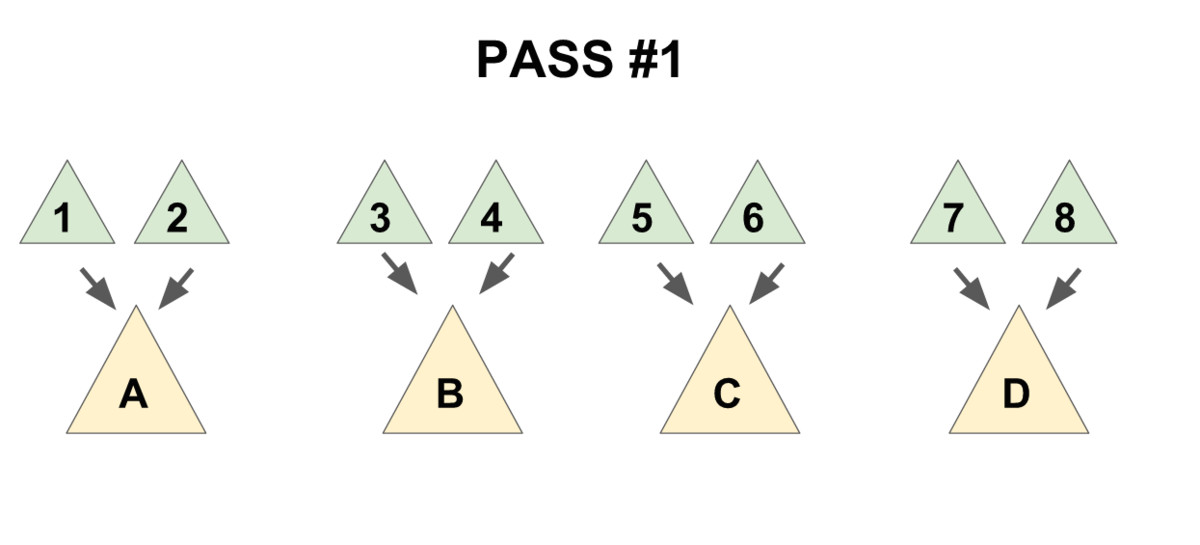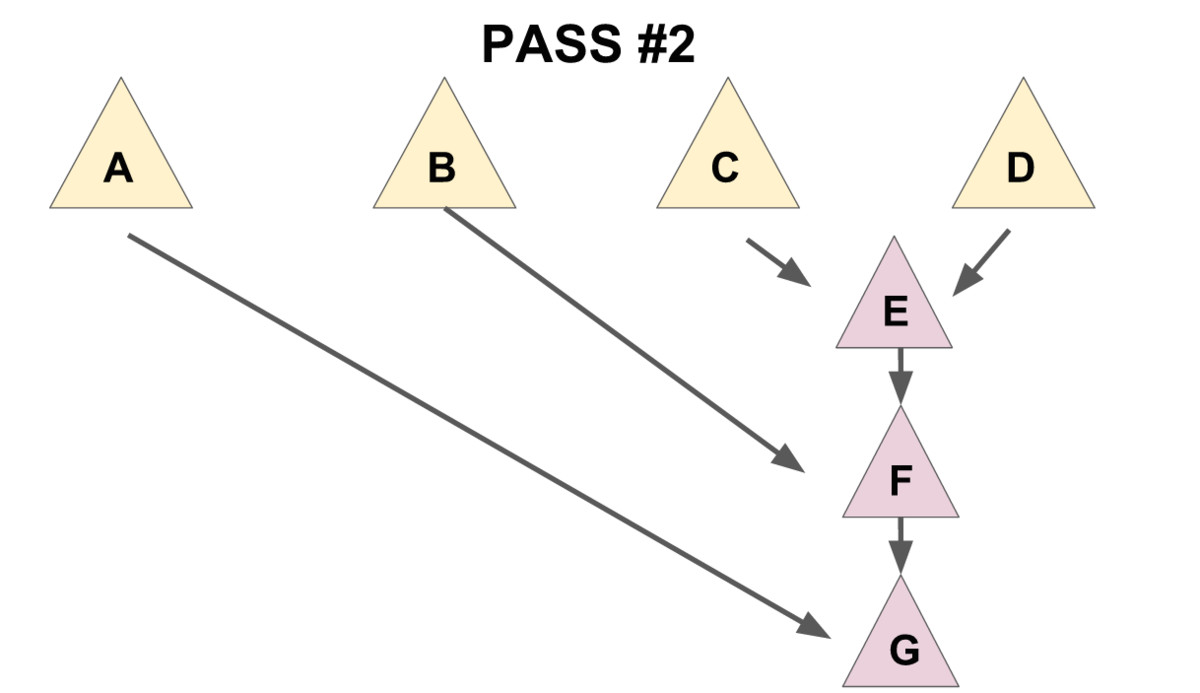# 配对堆

## 定义1 2 3 4 5 6 struct Node { T v; // T为权值类型 Node *child, *sibling; // child 指向该节点第一个儿子，sibling 指向该节点的下一个兄弟。 // 若该节点没有儿子/下个兄弟则指针指向 nullptr。 }; 

## 过程

### 合并

  1 2 3 4 5 6 7 8 9 10 Node* meld(Node* x, Node* y) { // 若有一个为空则直接返回另一个 if (x == nullptr) return y; if (y == nullptr) return x; if (x->v > y->v) std::swap(x, y); // swap后x为权值小的堆，y为权值大的堆 // 将y设为x的儿子 y->sibling = x->child; x->child = y; return x; // 新的根节点为 x } 

### 删除最小值

1. 把儿子们两两配成一对，用 meld 操作把被配成同一对的两个儿子合并到一起（见下图 1），
2. 将新产生的堆 从右往左（即老的儿子到新的儿子的方向）挨个合并在一起（见下图 2）。1 2 3 4 5 6 7 8 Node* merges(Node* x) { if (x == nullptr || x->sibling == nullptr) return x; // 如果该树为空或他没有下一个兄弟，就不需要合并了，return。 Node* y = x->sibling; // y 为 x 的下一个兄弟 Node* c = y->sibling; // c 是再下一个兄弟 x->sibling = y->sibling = nullptr; // 拆散 return meld(merges(c), meld(x, y)); // 核心部分 } 

1. meld(x,y)「配对」了 x 和 y。
2. merges(c) 递归合并 c 和他的兄弟们。
3. 将上面 2 个操作产生的 2 个新树合并。

 1 2 3 4 5 Node* delete_min(Node* x) { Node* t = merges(x->child); delete x; // 如果需要内存回收 return t; } 

### 减小一个元素的值

 1 2 3 4 5 6 struct Node { LL v; int id; Node *child, *sibling; Node *father; // 新增：父指针，若该节点为根节点则指向空节点 nullptr }; 

meld 操作修改为：

  1 2 3 4 5 6 7 8 9 10 11 12 Node* meld(Node* x, Node* y) { if (x == nullptr) return y; if (y == nullptr) return x; if (x->v > y->v) std::swap(x, y); if (x->child != nullptr) { // 新增：维护父指针 x->child->father = y; } y->sibling = x->child; y->father = x; // 新增：维护父指针 x->child = y; return x; } 

merges 操作修改为：

 1 2 3 4 5 6 7 8 9 Node *merges(Node *x) { if (x == nullptr) return nullptr; x->father = nullptr; // 新增：维护父指针 if (x->sibling == nullptr) return x; Node *y = x->sibling, *c = y->sibling; y->father = nullptr; // 新增：维护父指针 x->sibling = y->sibling = nullptr; return meld(merges(c), meld(x, y)); } 

  1 2 3 4 5 6 7 8 9 10 11 12 13 14 15 16 17 18 // root为堆的根，x为要操作的节点，v为新的权值，调用时需保证 v <= x->v // 返回值为新的根节点 Node *decrease_key(Node *root, Node *x, LL v) { x->v = v; // 更新权值 if (x == root) return x; // 如果 x 为根，则直接返回 // 把x从fa的子节点中剖出去，这里要分x的位置讨论一下。 if (x->father->child == x) { x->father->child = x->sibling; } else { x->father->sibling = x->sibling; } if (x->sibling != nullptr) { x->sibling->father = x->father; } x->sibling = nullptr; x->father = nullptr; return meld(root, x); // 重新合并 x 和根节点 }